Courses
Courses for Kids
Free study material
Offline Centres
More

# NCERT Solutions for Class 8 Maths Chapter 5 - ExerciseLast updated date: 27th Nov 2023
Total views: 528.6k
Views today: 15.28k

## NCERT Solutions for Class 8 Maths Chapter 5 Data Handling (EX 5.2) Exercise 5.2

Vedantu NCERT Solutions for Class 8 Maths Chapter 5 Exercise 5.2 have been designed to make students well-versed with the mathematical concepts that they can apply to accelerate in their careers. These NCERT solutions will help students solve their regular queries and persuade them to play with numbers. The NCERT solution of Class 8 Maths Chapter 5 will not only help you while doing your homework but will also help you in your exams through the step-wise solutions provided by Vedantu.

Science Students who are looking for NCERT Solutions for Class 8 Science will also find the Solutions curated by our Master Teachers really Helpful.

## Access NCERT Solutions for Class 8 Chapter 5 - Data Handling

Exercise 5.2

Refer to pages 6-7 for exercise 5.2 in the PDF

1. A survey was made to find the type of music that a certain group of young people liked in a city. The pie chart shows the findings of this survey. From this pie chart, answer the following: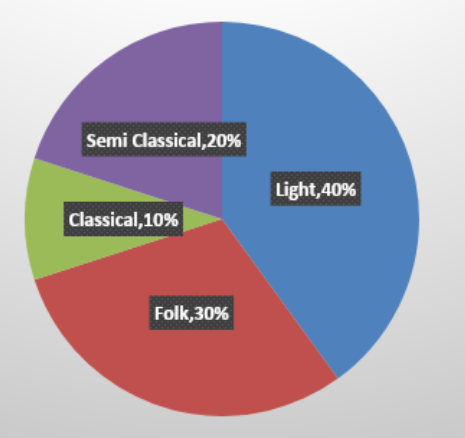Pie chart Representing type of Music

(i) If ${\mathbf{20}}$ people liked classical music, how many young people were surveyed?

Ans: In this part it is given that 20 people liked classical music and we have to find the total number of people who were surveyed.

So, from a given pie chart it is given that $10\%$ peoples like classical music.

Let the total number of people who were surveyed be $x$.

So, $10\%$ of $x$ must be equal to $20$.

That is,

$x \times \frac{{10}}{{100}} = 20$

$\frac{x}{{10}} = 20$

By cross multiplication we get,

$x = 200$

So, $200$ young people were surveyed.

(ii) Which type of music is liked by the maximum number of people?

Ans: In this part we have to find which type of music is liked by the maximum number of people.

So, from the given pie chart it is clear that light music is liked by the maximum number of people ($40\%$of the total number of people which is maximum in all categories).

(iii) If a cassette company were to make ${\mathbf{1000}}$ CDs, how many of each type would they make?

Ans: In this part it is given that if a cassette company were to make $100$ CDs, then how many of each type would they make?

For CDs of classical music:

Cassette Company has to make $10\%$CDs of classical music.

That is, $10\%$of the total number of CDs.

Let, $x$be the number of classical music CDs made by the company.

So,

$x = 1000 \times \frac{{10}}{{100}}$

$x = 100$

That is, the number of classical music CDs made by the company is$100$.

For CDs of semi classical music:

Cassette Company has to make $20\%$ CDs of semi classical music.

That is, $20\%$ of the total number of CDs.

Let, $y$ be the number of semi classical music CDs made by the company.

So, $y = 1000 \times \frac{{20}}{{100}}$

$y = 200$

That is, the number of semi classical music CDs made by the company is $200$.

For CDs of light music:

Cassette Company has to make $40\%$ CDs of light music.

That is, $40\%$of the total number of CDs.

Let, $z$ be the number of light music CDs made by the company.

So, $z = 1000 \times \frac{{40}}{{100}}$

$z = 400$

That is, the number of light music CDs made by the company is$400$.

For CDs of Folk music:

Cassette Company has to make $30\%$ CDs of Folk music.

That is, $30\%$of the total number of CDs.

Let $t$ be the number of Folk music CDs made by the company.

So, $t = 1000 \times \frac{{30}}{{100}}$

$t = 300$

That is, the number of Folk music CDs made by the company is $300$.

2. A group of ${\mathbf{360}}$ people were asked to vote for their favorite season from the three seasons: rainy, winter, and summer.

 Season No. of Votes Summer $90$ Rainy $120$ Winter $150$

(i) Which season got the most votes?

Ans: From the given table it is clear that winter season got the most votes

That is,$150$votes.

(ii) Find the central angle of each sector.

Ans: In this part we have to find the central angle of each sector. That is the angle made by each sector at the Centre of circle or pie chart.

So, the total number of votes is $360$.

Therefore, the central angle of summer season$= \frac{{90 \times {{360}^ \circ }}}{{360}}$

$= 90$

The central angle of rainy season $= \frac{{120 \times {{360}^ \circ }}}{{360}}$

$= {\text{ }}120$

The central angle of winter season $= \frac{{150 \times {{360}^ \circ }}}{{360}}$

$= {\text{ }}150$

(iii) Draw a pie chart to show this information.

Ans: To draw the pie chart we use the central angle which is calculated in the part (i) of this question.

The pie chart of the following data is as follows: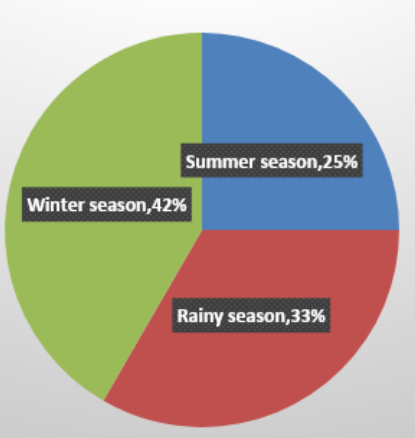Pie chart representing favorite seasons of people

3. Draw a pie chart showing the following information. The table shows the colours preferred by a group of people.

 Colours Number of People BlueGreenRedYellow ${\mathbf{18}}$0${\mathbf{6}}$${\mathbf{3}}$ Total ${\mathbf{36}}$

Ans: To draw the pie chart of the following information we have to calculate the central angle of each sector first.

Total central angle $= 360$

Total number of people $= {\text{ }}36$

So, the central angle of Blue colour$= \frac{{18 \times {{360}^ \circ }}}{{36}}$

$= {180^ \circ }$

The central angle of Green colour$= \frac{{9 \times {{360}^ \circ }}}{{36}}$

$= {90^ \circ }$

The central angle of Red colour$= \frac{{6 \times {{360}^ \circ }}}{{36}}$

$= {60^ \circ }$

The Central angle of Yellow colour$= \frac{{3 \times {{360}^ \circ }}}{{36}}$

$= {30^ \circ }$

The pie chart is as follows: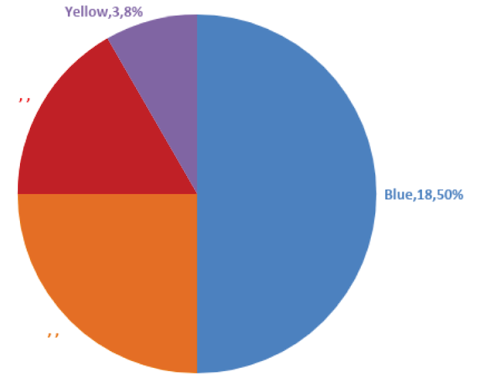Pie chart representing colours preferred by a group of people

4. The adjoining pie chart gives the marks scored in an examination by a student in Hindi, English, Mathematics, Social Science and Science. If the total marks obtained by the students were${\mathbf{540}}$, answer the following questions: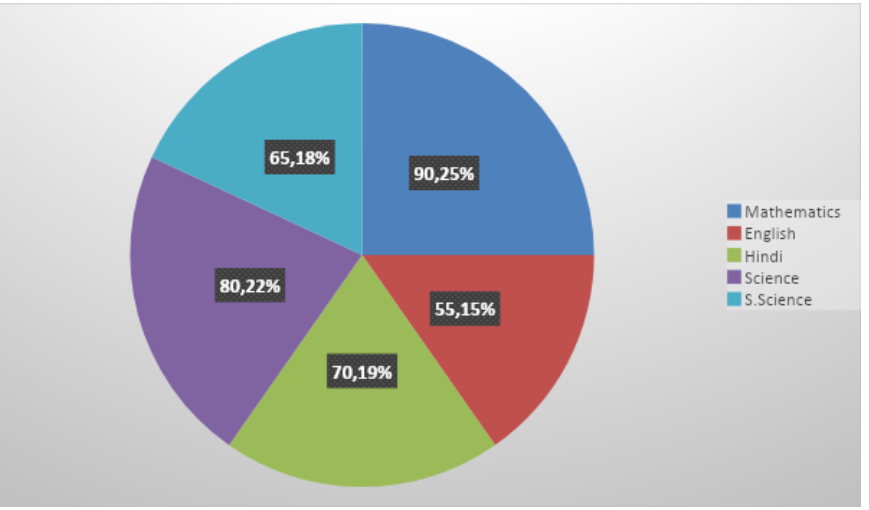Pie chart representing the marks scored

(i) In which subject did the student score ${\mathbf{105}}$ marks?

(Hint: for ${\mathbf{540}}$ marks, the central angle =${\mathbf{360}}$. So, for ${\mathbf{105}}$ marks, what is the central angle?)

Ans: Given, Total marks$= 540$.

And we know that the central angle$= 360^ \circ$.

So, the central angle made by the sector having 105 marks$= \frac{{105 \times {{360}^ \circ }}}{{540}}$

$= {70^ \circ }$

Therefore, In Hindi students score $105$ marks.

(ii) How many more marks were obtained by the student in Mathematics than in Hindi?

Ans: From the previous part we know that the student scores $105$ marks in Hindi.

Now we have to calculate the marks in Mathematics.

We know that the central angle made by the Mathematics sector$= {90^ \circ }$.

And the total scored marks$= 540$.

Let the marks scored in Mathematics be $x$.

Therefore,

$\frac{{x \times {{360}^ \circ }}}{{540}} = {90^ \circ }$

$x = \frac{{90 \times 54}}{{36}}$

So,

$x = 135$

Now, the difference of marks scored in Mathematics and Hindi $= {\text{ }}30$.

So, the student obtained $30$ more marks in Mathematics than in Hindi.

(iii) Examine whether the sum of the marks obtained in Social Science and Mathematics is more than that in Science and Hindi.

(Hint: Just study the central angles)

Ans: From the previous parts we know that the student score $105$ marks in Hindi and $135$ marks in Mathematics.

Now we have to calculate the marks in Science and Social Science.

For Science:

We know that the central angle made by the Science sector $= {80^ \circ }$.

And the total scored marks $= 540$.

Let the marks scored in science be y.

Therefore,

$\frac{{y \times 360}}{{540}} = 80$

$y = \frac{{80 \times 54}}{{36}}$

So, $y = 120$

That is, students score $120$ marks in science.

For Social Science:

As we know that the central angle is made by the Social Science sector $= {65^ \circ }$.

And the total scored marks $= 540$.

Let the marks scored in Social Science be $y$.

Therefore,

$\frac{{y \times 360}}{{540}} = 65$

$y = \frac{{65 \times 54}}{{36}}$

So,

$y = 97.5$

That is, student score $97.5$ marks in Social Science.

Now, the sum of the marks scored in Social Science and Mathematics $= 97.5 + 135$

$= 232.5$

And the sun of the marks scored in Science and Hindi $= {\text{ }}120 + 105$

$= {\text{ }}225$

Therefore, the sum of the marks obtained in Social Science and Mathematics is more than that in Science and Hindi.

5. The number of students in a hostel, speaking different languages is given below. Display the data in a pie chart.

 Language Hindi English Marathi Tamil Bengali Total No. of Students ${\mathbf{40}}$ ${\mathbf{12}}$ ${\mathbf{9}}$ ${\mathbf{7}}$ ${\mathbf{4}}$ ${\mathbf{72}}$

Ans: To draw the pie chart of the given data. First we have to find the central angle of each sector.

Given, the total number of students $= {\text{ }}72$.

The total central angle $= {360^ \circ }$

Now, calculating the central angle of each sector.

For Hindi:

Central angle of Hindi $= \frac{{40 \times 360}}{{72}}$

So, the central angle of Hindi $= 200$

For English:

Central angle of English $= \frac{{12 \times 360}}{{72}}$

So, the central angle of English $= 60$

For Marathi:

Central angle of Marathi $= \frac{{9 \times 360}}{{72}}$

So, the central angle of Marathi $= 45$

For Tamil:

Central angle of Tamil $= \frac{{7 \times 360}}{{72}}$

So, the central angle of Tamil $= 35$

For Bengali:

Central angle of Bengali $= \frac{{4 \times 360}}{{72}}$

So, the central angle of Bengali$= 20$

Now, the pie chart of the following data is as follows: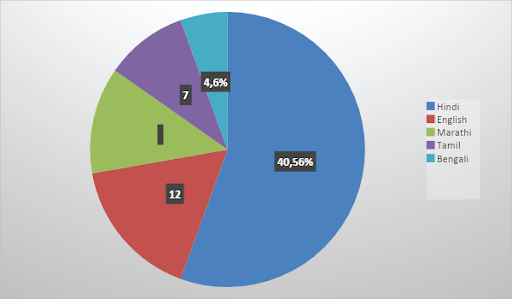Pie chart representing different languages spoken

## Benefits of NCERT solution of Class 8Maths Chapter 5 Exercise 5.2

The Vedantu solutions have been designed to give you an overall view of the chapter, along with the precise answer to all your mathematical problems. Vedantu solutions have to date helped millions of students achieve their target score by teaching them to apt format and the exact steps that attract marks in exams. Few of the many benefits that come along with the Vedantu solutions include:

NCERT solution Class 8 Maths Chapter 5 Exercise 5.2 talks to the point and provides precisely what is desired. Along with every question, the steps are thoroughly explained, and the reasons are also mentioned as to why a specific formula is used in the solution. The biggest reason why Vedantu is so preferred that it provides solutions in a very sorted manner. All the solutions are available chapter-wise in pdf manner on the internet. These are easy to download and easy to save for further use too.

All the high-quality answers have been provided by the experts who are closely associated with NCERT and have developed top-class acumen in the same. Maths NCERT questions Class 8Chapter 5 Exercise 5.2 might be challenging, but Maths NCERT Solutions Class 8 Chapter 5 Exercise 5.2 by Vedantu are certainly not. The detailed notes will help you not skip any point and score well in exams. Vedantu reaches only to experienced teachers who provide easy solutions to all problems. Even if something new is introduced in any question, it comes with apparent reason and back story explaining its application and use. To top it all, Vedantu does not only provide solutions to your NCERT math problems but also gives some sample questions that match the NCERT style entirely to let you practice and prepare well for the competition. All the solutions provided for NCERT Maths Class 8 Chapter 5 Exercise 5.2 adhere to the NCERT instructions and do not teach anything that is not apt, according to NCERT.

Vedantu offers a user-friendly interface so that it is easy for people of all ages to learn through Vedantu. To make learning even fun and challenging, Vedantu also provides in between tests. These tests are again designed according to NCERT and have been approved by the experts. The students who have written these tests claim that they helped them in their exams. Vedantu is also of help to students who missed their classes in the past because of some reasons because there isn’t any topic that is left untouched in Class 8th Maths Chapter 5 Exercise 5.2. Vedantu discusses even the minor things that are sometimes skipped in the classroom. Not only this, but you can locate solutions to your other math problems also on Verdantu by typing easy keywords.

### Why choose Vedantu?

In the era of technology, learning is fun and accessible through the internet. You can easily find the exact solution that you are looking for. Vedantu provides its users with all the desired answers in one place, which requires them to navigate the least and also saves time and energy. The fact that experts authorize all the answers makes the chances of them being wrong minimal. Vedantu makes use of specialized tools and techniques that make learning exciting and exciting for kids. Also, Vedantu is very easy to use.

So, what are you waiting for? Join Vedantu now and start learning! You can either sign up on the web portal of Vedantu or make learning even more easy for you; Vedantu has also launched an app, which means learning is just on your fingertips now.